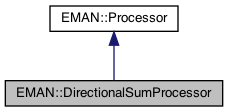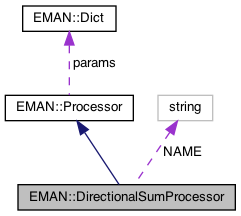EMAN2
EMAN::DirectionalSumProcessor Class Reference

Does a projection in one the axial directions Doesn't support process_inplace (because the output has potentially been compressed in one dimension) More...

`#include <processor.h>`

Inheritance diagram for EMAN::DirectionalSumProcessor:[legend]
Collaboration diagram for EMAN::DirectionalSumProcessor:[legend]

## Public Member Functions

virtual string get_name () const
Get the processor's name. More...

virtual EMDataprocess (const EMData *const image)

virtual void process_inplace (EMData *)

virtual TypeDict get_param_types () const
Get processor parameter information in a dictionary. More...

string get_desc () const
Get the descrition of this specific processor. More...Public Member Functions inherited from EMAN::Processor
virtual ~Processor ()

virtual void process_list_inplace (vector< EMData * > &images)
To process multiple images using the same algorithm. More...

virtual Dict get_params () const
Get the processor parameters in a key/value dictionary. More...

virtual void set_params (const Dict &new_params)
Set the processor parameters using a key/value dictionary. More...

## Static Public Member Functions

static ProcessorNEW ()Static Public Member Functions inherited from EMAN::Processor
static string get_group_desc ()
Get the description of this group of processors. More...

static void EMFourierFilterInPlace (EMData *fimage, Dict params)
Compute a Fourier-filter processed image in place. More...

static EMDataEMFourierFilter (EMData *fimage, Dict params)
Compute a Fourier-processor processed image without altering the original image. More...

## Static Public Attributes

static const string NAME = "misc.directional_sum"Public Types inherited from EMAN::Processor
enum  fourier_filter_types {
TOP_HAT_LOW_PASS , TOP_HAT_HIGH_PASS , TOP_HAT_BAND_PASS , TOP_HOMOMORPHIC ,
GAUSS_LOW_PASS , GAUSS_HIGH_PASS , GAUSS_BAND_PASS , GAUSS_INVERSE ,
GAUSS_HOMOMORPHIC , BUTTERWORTH_LOW_PASS , BUTTERWORTH_HIGH_PASS , BUTTERWORTH_HOMOMORPHIC ,
KAISER_I0 , KAISER_SINH , KAISER_I0_INVERSE , KAISER_SINH_INVERSE ,
SHIFT , TANH_LOW_PASS , TANH_HIGH_PASS , TANH_HOMOMORPHIC ,
}
Fourier filter Processor type enum. More...Protected Attributes inherited from EMAN::Processor
Dict params

## Detailed Description

Does a projection in one the axial directions Doesn't support process_inplace (because the output has potentially been compressed in one dimension)

Parameters
 direction The direction of the sum, either x,y or z

Definition at line 7646 of file processor.h.

## ◆ get_desc()

 string EMAN::DirectionalSumProcessor::get_desc ( ) const
inlinevirtual

Get the descrition of this specific processor.

This function must be overwritten by a subclass.

Returns
The description of this processor.

Implements EMAN::Processor.

Definition at line 7680 of file processor.h.

7681 {
7682 return "Calculates the projection of the image along one of the axial directions, either x, y or z";
7683 }

## ◆ get_name()

 virtual string EMAN::DirectionalSumProcessor::get_name ( ) const
inlinevirtual

Get the processor's name.

Each processor is identified by a unique name.

Returns
The processor's name.

Implements EMAN::Processor.

Definition at line 7649 of file processor.h.

7650 {
7651 return NAME;
7652 }
static const string NAME
Definition: processor.h:7685

References NAME.

## ◆ get_param_types()

 virtual TypeDict EMAN::DirectionalSumProcessor::get_param_types ( ) const
inlinevirtual

Get processor parameter information in a dictionary.

Each parameter has one record in the dictionary. Each record contains its name, data-type, and description.

Returns
A dictionary containing the parameter info.

Reimplemented from EMAN::Processor.

Definition at line 7671 of file processor.h.

7672 {
7673 TypeDict d;
7674 d.put("axis", EMObject::STRING,"The direction of the sum, either x,y or z. Returned axes are xy, xz or zy.");
7675 d.put("first", EMObject::INT,"The first position along the speficied axis to use in the sum. Neg val -> nx/y/z+first (default=0)");
7676 d.put("last", EMObject::INT,"The last position along the speficied axis to use in the sum. Neg val -> nx/y/z+last (default=-1)");
7677 return d;
7678 }
TypeDict is a dictionary to store <string, EMObject::ObjectType> pair.
Definition: emobject.h:305
void put(const string &key, EMObject::ObjectType o, const string &desc="")
Definition: emobject.h:330

References EMAN::EMObject::INT, EMAN::TypeDict::put(), and EMAN::EMObject::STRING.

## ◆ NEW()

 static Processor * EMAN::DirectionalSumProcessor::NEW ( )
inlinestatic

Definition at line 7654 of file processor.h.

7655 {
7656 return new DirectionalSumProcessor();
7657 }
Does a projection in one the axial directions Doesn't support process_inplace (because the output has...
Definition: processor.h:7647

## ◆ process()

 EMData * DirectionalSumProcessor::process ( const EMData *const image )
virtual
Exceptions
 InvalidParameterException raised if the direction parameter is not "x", "y" or "z"

Reimplemented from EMAN::Processor.

Definition at line 8811 of file processor.cpp.

8811 {
8812 string dir = params.set_default("axis", "");
8813 if ( dir == "" || ( dir != "x" && dir != "y" && dir != "z" ) )
8814 throw InvalidParameterException("The direction parameter must be either x, y, or z");
8815
8816 int nx = image->get_xsize();
8817 int ny = image->get_ysize();
8818 int nz = image->get_zsize();
8819 EMData* ret = new EMData;
8820 if (image->has_attr("ptcl_repr")) ret->set_attr("ptcl_repr",image->get_attr("ptcl_repr"));
8821 if (image->has_attr("xform_align2d")) ret->set_attr("xform_align2d",image->get_attr("xform_align2d"));
8822 if (image->has_attr("xform_align3d")) ret->set_attr("xform_align3d",image->get_attr("xform_align3d"));
8823 ret->set_attr("apix_y",image->get_attr("apix_y")); // potentially meaningless, may get overwritten, but better to be self-consistent
8824 ret->set_attr("apix_z",image->get_attr("apix_z")); // meaningless, but better to be self-consistent
8825
8826
8827 if (nz==1) {
8828 if (dir=="x") {
8829 ret->set_size(ny,1,1);
8830 ret->set_attr("apix_x",image->get_attr("apix_y"));
8831 for (int y=0; y<ny; y++) {
8832 double sm=0;
8833 for (int x=0; x<nx; x++) sm+=image->get_value_at(x,y);
8834 ret->set_value_at(y,0,0,sm/nx);
8835 }
8836 return ret;
8837 }
8838 else if (dir=="y") {
8839 ret->set_size(nx,1,1);
8840 ret->set_attr("apix_x",image->get_attr("apix_x"));
8841 for (int x=0; x<nx; x++) {
8842 double sm=0;
8843 for (int y=0; y<ny; y++) sm+=image->get_value_at(x,y);
8844 ret->set_value_at(x,0,0,sm/ny);
8845 }
8846 return ret;
8847 }
8848 else throw InvalidParameterException("The direction parameter must be either x, y for 2-D images");
8849 }
8850
8851 int a0 = params.set_default("first", 0);
8852 int a1 = params.set_default("last", -1);
8853
8854 // compress one of the dimensions
8855 if ( dir == "x" ) {
8856 ret->set_size(nz,ny);
8857 ret->set_attr("apix_x",image->get_attr("apix_z"));
8858 ret->set_attr("apix_y",image->get_attr("apix_y"));
8859
8860 // bounds checks
8861 if (a0<0) a0+=nx;
8862 if (a1<0) a1+=nx;
8863 if (a0<0) a0=0;
8864 if (a1<0) a1=0;
8865 if (a0>=nx) a0=nx-1;
8866 if (a1>=nx) a1=nx-1;
8867
8868 for (int y=0; y<ny; y++) {
8869 for (int z=0; z<nz; z++) {
8870 double sum=0.0;
8871 for (int x=a0; x<=a1; x++) sum+=image->get_value_at(x,y,z);
8872 ret->set_value_at(z,y,(float)sum);
8873 }
8874 }
8875 }
8876 else if ( dir == "y" ) {
8877 ret->set_size(nx,nz);
8878 ret->set_attr("apix_x",image->get_attr("apix_x"));
8879 ret->set_attr("apix_y",image->get_attr("apix_z"));
8880
8881 // bounds checks
8882 if (a0<0) a0+=ny;
8883 if (a1<0) a1+=ny;
8884 if (a0<0) a0=0;
8885 if (a1<0) a1=0;
8886 if (a0>=ny) a0=ny-1;
8887 if (a1>=ny) a1=ny-1;
8888
8889 for (int x=0; x<nx; x++) {
8890 for (int z=0; z<nz; z++) {
8891 double sum=0.0;
8892 for (int y=a0; y<=a1; y++) sum+=image->get_value_at(x,y,z);
8893 ret->set_value_at(x,z,(float)sum);
8894 }
8895 }
8896 }
8897 else if ( dir == "z" ) {
8898 ret->set_size(nx,ny);
8899 ret->set_attr("apix_x",image->get_attr("apix_x"));
8900 ret->set_attr("apix_y",image->get_attr("apix_y"));
8901
8902 // bounds checks
8903 if (a0<0) a0+=nz;
8904 if (a1<0) a1+=nz;
8905 if (a0<0) a0=0;
8906 if (a1<0) a1=0;
8907 if (a0>=nz) a0=nz-1;
8908 if (a1>=nz) a1=nz-1;
8909
8910 for (int y=0; y<ny; y++) {
8911 for (int x=0; x<nx; x++) {
8912 double sum=0.0;
8913 for (int z=a0; z<=a1; z++) sum+=image->get_value_at(x,y,z);
8914 ret->set_value_at(x,y,(float)sum);
8915 }
8916 }
8917 }
8918
8919 ret->update();
8920 return ret;
8921}
type set_default(const string &key, type val)
Default setting behavior This can be achieved using a template - d.woolford Jan 2008 (before there wa...
Definition: emobject.h:569
EMData stores an image's data and defines core image processing routines.
Definition: emdata.h:82
#define InvalidParameterException(desc)
Definition: exception.h:361
#define y(i, j)
Definition: projector.cpp:1516
#define x(i)
Definition: projector.cpp:1517

References InvalidParameterException, EMAN::Processor::params, EMAN::Dict::set_default(), x, and y.

## ◆ process_inplace()

 virtual void EMAN::DirectionalSumProcessor::process_inplace ( EMData * )
inlinevirtual
Exceptions
 InvalidCallException raised if this function is called

Implements EMAN::Processor.

Definition at line 7667 of file processor.h.

7667 {
7668 throw InvalidCallException("The directional sum processor does not work inplace");
7669 }
#define InvalidCallException(desc)
Definition: exception.h:348

References InvalidCallException.

## ◆ NAME

 const string DirectionalSumProcessor::NAME = "misc.directional_sum"
static

Definition at line 7685 of file processor.h.

Referenced by get_name().

The documentation for this class was generated from the following files: